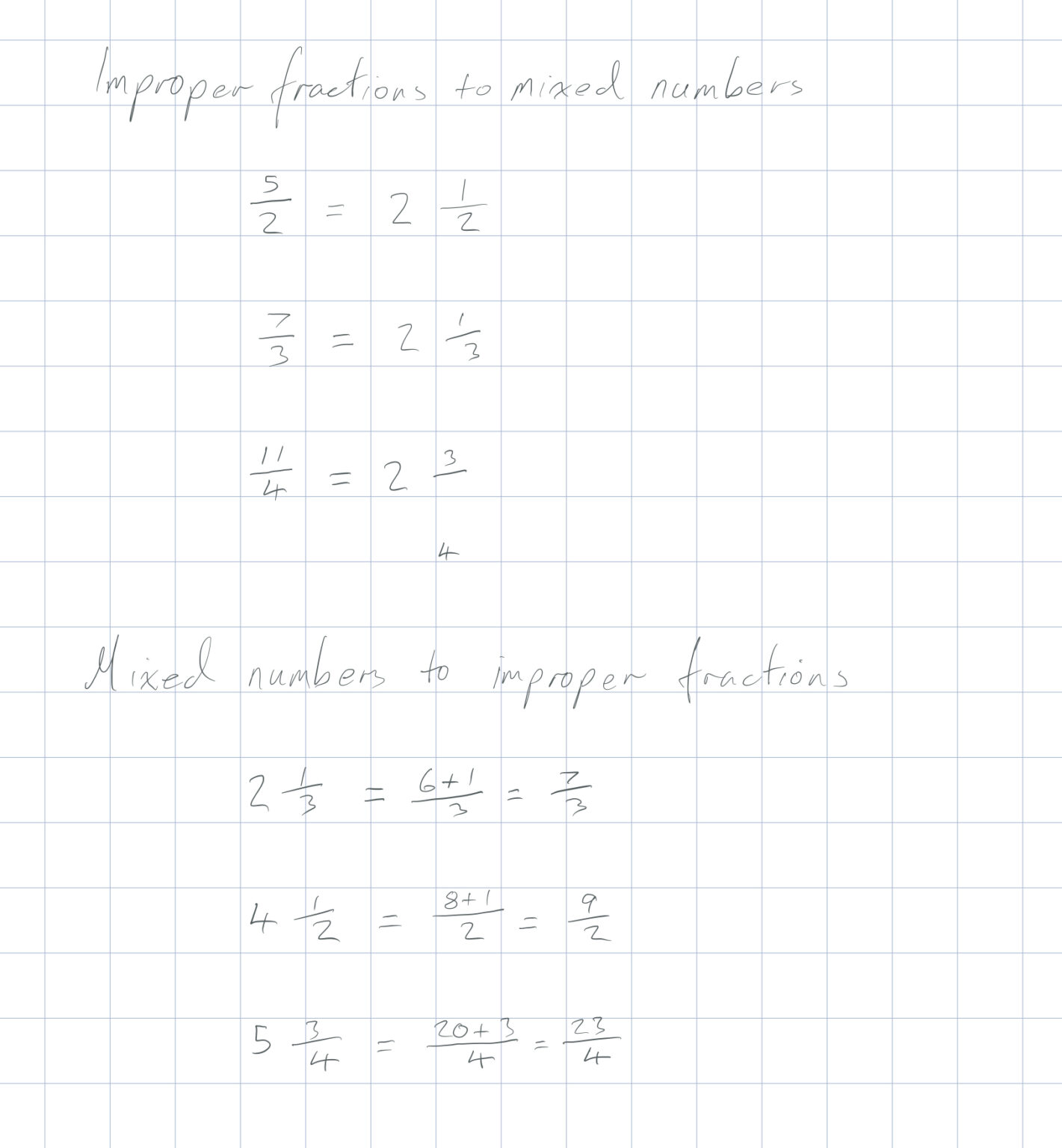Improper fractions are fractions with a larger number on the numerator (top number) than the denominator (bottom number).

Mixed Numbers are numbers with a whole number part and a fractional part.

To change from improper fractions to mixed numbers: divide the top number by the bottom number. The answer is the whole number and the remainder is what is left in the fraction.

To change from mixed numbers to improper fractions: multiply the whole number by the bottom number of the fraction and add it to the top number.